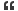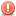# Thread: How many times the while loop execute

1. ## How many times the while loop execute

define void main(),int main()?what are the differences between void main() and int main(). int main()
{
int x=0;
while(x<10)
{
printf("%d", x);
x++;
}
how many times the while loop executeReply With Quote

2. ## Re: How many times the while loop executeOriginally Posted by chandanavemulapallydefine void main(),int main()?what are the differences between void main() and int main(). int main()
{
int x=0;
while(x<10)
{
printf("%d", x);
x++;
}
how many times the while loop execute
You have two questions. Lets tackle this one by one.

1.
main() is just like any other function in C. This means that it can take input arguments and also return a result value. So, the real declaration of main() is:
<return value > main (arg1, arg2, arg3...)

By specifying void main(), you are actually saying that main() will not return any value. By specifying int main(), you are saying that main() will return an integer value. The UNIX convention is that a return value of zero is success and a non-zero value indicates some failure code. Also, this return code from main can be examined by the shell to see if the program was successful (ret value of zero) or failure (non-zero return value). The non-zero return value can be successfully used as a debugging aid if the program returns different failure codes for different errors.

2.
The while loop will execute 10 times: from z=0 to x=9. When x becomes 10, it will bail out of the while loop. Why do you ask this question?? I mean to say: what doubt did you have that you asked this question??Reply With Quote

3. ## Re: How many times the while loop execute

void main()
the above method doesnot return any value.
int main()
the above method must return int value.
int main()
{
//statements
......
......
return 0;(may be placed or not)
}
the above program 10 times to be executedReply With Quote

4. ## Re: How many times the while loop execute

main() must return int. Not void, not bool, not float.Some compilers accept void main(), but that is non-standard and shouldn't be used. Instead use int main(). The while loop execute 10 times.Reply With Quote

5. ##Re: How many times the while loop execute

you have posted two questions so i want to answer one by one.
1:the difference between void main() and int main()
first of all we want to differentiate between both of them.
both of these are return type which are return some value to the os.

int to return anint valu to the os.

2->the while loop is executed ten times from 0to 9.that is 10 times.Reply With Quote

6. ## Re: How many times the while loop execute

yes the loop will be executed for x= 0 to x=9 and for 10 it will teminate

so it will execute 10 time

well we write void main() on the assumption that our main function isnt goin to return any value and in case of int main() the main function is supposed to return an integer value

thus within an int main() function we shud have the habbit of writing return 1 at last which is an indication that the fucntion will return an intger valueReply With Quote

7. ## Re: How many times the while loop executeOriginally Posted by chandanavemulapallydefine void main(),int main()?what are the differences between void main() and int main(). int main()
{
int x=0;
while(x<10)
{
printf("%d", x);
x++;
}
how many times the while loop execute

it will execute for 10 times from 0to9Reply With Quote

8. ## Re: How many times the while loop execute

As sumone above has given an answer tat main can be declared as like as other function den it's contradictory coz declaration of main is done by the compiler called by the operating system definition is given by the programmer . Diff btw void n int main is tat in the former no value will be returned where as in the latter value will be returned if v don mention ny return type den a garbage value will be returned......Reply With Quote

9.

####Posting Permissions

• You may not post new threads
• You may not post replies
• You may not post attachments
• You may not edit your posts
•